## [Serial Communication Excercise] Cole and Minh

Exercise 1: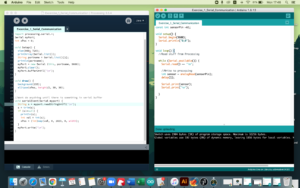Excercise 2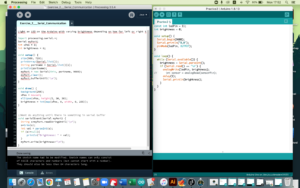Exercise 3:

```const int ledPin = 11;
int brightness = 0;
const int sensorPin = A0;

void setup() {
Serial.begin(9600);
Serial.println("0,0");
pinMode(ledPin, OUTPUT);
}

void loop() {
while (Serial.available()) {
int onOff = Serial.parseInt();
digitalWrite(ledPin, onOff);
delay(1);
Serial.println(sensor);
}
}
}

//PROCESSING CODE
velocity.mult(drag);
acceleration.mult(0);
ellipse(position.x,position.y,mass,mass);
fill(255,255,0);
if (position.y > height-mass/2) {
velocity.y *= -0.9;  // A little dampening when hitting the bottom
position.y = height-mass/2;
onOff = true;
}
else{
onOff = false;
}
}

void serialEvent(Serial myPort){
s=trim(s);
int val = parseInt(s);
if (s!=null){
wind.x=map(val,0,1023,-2, 2);  //2 instead of 1 to make it stronger
}
myPort.write(int(onOff)+"\n");
}

void applyForce(PVector force){
// Newton's 2nd law: F = M * A
// or A = F / M
PVector f = PVector.div(force, mass);
}

void keyPressed(){
if (keyCode==LEFT){
wind.x=-1;
}
if (keyCode==RIGHT){
wind.x=1;
}
if (key==' '){
mass=random(15,80);
position.y=-mass;
velocity.mult(0);
}
}```

Video for exercise 3:

For this week’s assignment, we are asked to use at least one analog input to control one LED and at least one digital input to control one LED. I haven’t added the sketch yet so I am going to come back and add that creative element later!

I had fun with the line graph displaying the knobValue before and after being mapped. I made the graph curve by rotating the potentiometer. Below are the graph and the video of that: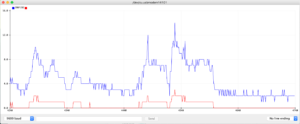This is the code (how to make it color-coded though?) (updated: oh! it is color-coded in the post but not right in the editing space)

```const int ledPinYellow = 3;
const int ledPinGreen = 7;
const int ledPinBlue = 12;
const int buttonPin = 2;
int knobPin = A0;
int ledState = LOW;
int prevButtonState = LOW;

void setup() {
pinMode(ledPinYellow, OUTPUT);
pinMode(ledPinGreen, OUTPUT);
pinMode(ledPinBlue, OUTPUT);
pinMode(buttonPin, INPUT);
//pinMode(A0, INPUT);

Serial.begin(9600);
}

void loop() {
int mappedValue = map(knobValue, 0, 1023, 0, 255);
Serial.print(knobValue);
Serial.print("    ");
Serial.println(mappedValue);

analogWrite(ledPinYellow, mappedValue);

if (currentButtonState == HIGH && prevButtonState == LOW) {
// flip the LED state
if (ledState == HIGH){
ledState = LOW;
} else if (ledState == LOW){
ledState = HIGH;
}
}
// if you want to print out the LED state
// Serial.print(ledState);

digitalWrite(ledPinGreen, ledState);
digitalWrite(ledPinBlue, !ledState);

//we need to remember the state of our button for the next time through LOOP
prevButtonState = currentButtonState;
}```

## Midterm Assignment: Flying Campus Cat

Motivation

I want to replicate the game Flappy Bird but with some modifications in choosing the image and adding some sounds to it such as the background music and the cat’s sound when it is alive/dead.

Process

The game requires player to click mouse to move the cat upwards. If the mouse is not pressed, the cat will be falling down. By controlling the direction and the velocity of the cat, player needs to avoid hitting the palm trees which approach the cat from the right hand side. The final score is the number of times the cat flies through 2 palm tress.

I encountered first problems when trying to upload the sound file to Processing library. It seems Processing on my laptop cannot read the tail .mp3 so I had to have all the mp3 files converted to wav files. The example from processing.org uses mp3 and it works but not for my laptop. I think it can be related to the version of the software. Regarding the class Palm Tree, I used a not so technical method to draw the foliage. I drew a lot of triangles and put one on top of the other to (hopefully) give it a look like the palm tree. Firstly, I drew a palm tree using only triangles on a coordinate plane to check the coordinates of all the points I needed. Then I used Excel to organize all the numbers a bit and gradually form triangles by grouping 3 points together.

Code

Palm tree class

```// Draw the palm tree
public class palmTree {
void draw(float x, float y, boolean flip){
smooth();
noStroke();

// Turn the palm trees upside down to create 2 lines of obstacles on both sides
int flipper = 1;
if (flip) flipper = -1;

//Draw the tree trunk
fill(94,62,28);
//rect (0 + x,0 + y,50 + x,-200+y);
rect(x, y, 50, -1000*flipper);

//Draw the foliage by putting a lot of triangles on top of each other.
//The numbers were collected from a mobile app allowing users to know the coordinates of each point when a random shape is drawn
fill(62, 145, 32);
triangle(50 + x,  y, 0 + x, (57.5)*flipper + y, -39.25 + x,  (19.25)*flipper + y);
triangle(50 + x, y,-39.25 + x, (88.75)*flipper + y, 49.5 + x,  (62.5)*flipper + y);
triangle(50 + x, y, 49.5 + x, (62.5)*flipper + y, 86.25 + x, (11.75)*flipper + y);
triangle(0 + x, y, 49.5 + x, (62.5)*flipper + y, 75 + x, (-40)*flipper + y);
triangle(50 + x, y, 0 + x, (57.5)*flipper + y, 57.5 + x, (107.5)*flipper + y);
triangle(0 + x, y, 0 + x, (57.5)*flipper + y, 90.75 + x, (76)*flipper + y);
triangle(50 + x, y, 49.5 + x, (62.5)*flipper + y, -2 + x, (110)*flipper + y);
triangle(50 + x, y, 0 + x, (57.5)*flipper + y, -30.5 + x, (-37.5)*flipper + y);
fill(0);
}

}```

Main class

```import processing.sound.*;

// Image files
PImage background;
PImage cat;

// Sound files
SoundFile backgroundMusic;
SoundFile catAlive;

palmTree pt;
PFont f;

int interfaceState = 1;
int point = 0;
int max = 0;
int x = -200, y;
int catFallingSpeed = 0;
int treeX[] = new int;
int treeY[] = new int;

void setup() {
size(800,600);
fill(0);
textSize(40);

cat.resize(0,70);

backgroundMusic = new SoundFile(this,"jazz.wav");
backgroundMusic.play();
catAlive = new SoundFile(this, "catAlive.wav");

pt = new palmTree();
}

int closestPillar = 0;

void draw() {

if(interfaceState == 0) {
imageMode(CORNER);
image(background, x, 0);
image(background, x+background.width, 0);

//Make the background move to create the illusion that the cat is flying forwards
x -= 5;
catFallingSpeed += 1;
y += catFallingSpeed;

if(x <= -background.width) x = 0;
for(int i = 0 ; i < 2; i++) {
imageMode(CENTER);

//Create the gap between two trees
pt.draw(treeX[i], treeY[i] - 150, false);
pt.draw(treeX[i], treeY[i] + 150, true);

if(treeX[i] < 0) {
treeY[i] = (int)random(200, height-200);
treeX[i] = width;
//point++;
}
if (treeX[i] < width/2 && i == closestPillar) {
max = max(++point, max);
closestPillar = 1 - closestPillar;}

//Conditions when the game ends including reaching the boundaries or colliding with the palm tree blocks
if (y > height || y < 0 || (abs(width/2-treeX[i])<25 && abs(y-treeY[i])>100)) interfaceState=1;
treeX[i] -= 5;
}
image(cat, width/2, y);
text(""+point, 20, 50);
}
else if (interfaceState==1) {
}
imageMode(CENTER);
image(background, width/2, height/2);
text("High Score: "+max, 50, width/2);
f = createFont("Courier New", 30);
textFont(f);
}
}

void mousePressed() {
catAlive.play();
catFallingSpeed = -17;
if(interfaceState==1) {
treeX = width;
treeY = y = height/2;
treeX = width*3/2;
treeY = 300;
x = interfaceState = point = 0;
}
}
```

Final game (I cannot record the sound of it)

Rooms for improvement

In the game, as long as the cat does now hit the tree trunk which is in a rectangular shape, the cat is still alive and the player can keep playing. I do not know how to check if the cat hits the spiky shape of the foliage to end the game at that point so I use collision with the tree trunk only as a determiner if the game is still ok or should be ended.

Also, I did not loop the background music so if a player is playing so well and exceed the time duration of around 2 minutes (the length of the track), the background music disappears.

The file containing all related assets can be accessed here: flying_campus_cat

After making the game and listening to the cat sound several times when testing it, I walked out seeing a cat and it hit differently :<

## Week 4: Shivering Letters

```//a string of text
String s = "Shivering in Abu Dhabi";
// Create an array the same size as the length of the String
Letter[] letters = new Letter[s.length()];
//Check the font list
//println(PFont.list());
PFont f;

void setup() {
size(600, 600);
f = createFont("Courier New", 30);
textFont(f);

// The initial position
int x = 100;
for (int i = 0; i < s.length (); i++) {
letters[i] = new Letter(x, height/2, s.charAt(i));
x += textWidth(s.charAt(i));
}
}

void draw() {
background(255,255,0);

// loop through the letters array and display letters
for (int i = 0; i < letters.length; i++) {
letters[i].display();

// If the mouse is pressed the letters shiver; otherwise, they return to their original location or station
if (mousePressed) {
letters[i].shiver();
} else {
letters[i].station();
}
}
}

```
```class Letter {
float x,y;
float X,Y;
float fluctuation;
char letter;

Letter (float _x, float _y, char _c) {
X = x = _x;
Y = y = _y;
x = random(width);
y = random(height);
fluctuation = random(TWO_PI);
letter = _c;
}

void display() {
//Choose random color for each letter
fill(random(255));
//strokeWeight(2);
textAlign(CENTER);
textSize(30);
pushMatrix();
translate(x,y);
rotate(fluctuation);
text(letter,0,0);
popMatrix();
}

// Fluctuate the letters
void shiver() {
x += random(-5,5);
y += random(-5,5);
fluctuation += random(-1,1);
}

// Return the letter to the initial positions using lerp function
void station() {
x = lerp(x,X,0.05);
y = lerp(y,Y,0.05);
fluctuation = lerp(fluctuation,0,0.05);
}
}```

Motivation

I want to display letters in a shaky form representing how tremendously the temperature fluctuates between daytime and night time in Abu Dhabi. I can wear sweaters at night or early morning and shorts at noon.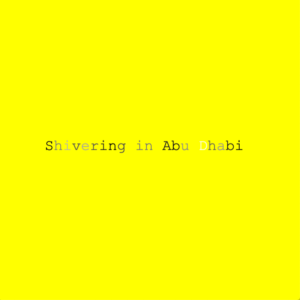Process

I want to make the letter blink in different shades of one color (I should have chosen red!) to represent the temperature change. When the mouse is pressed, individual letters moved freely around the initial position and they come back to the station and stand still when the mouse is released. Also, I based the code for my class Letter on  our class file circleLetterSketch and took reference from The Coding Train Youtube channel. In the coding and googling process, I discovered the println(PFont.list()) funtion to know all the fonts available in Processing after some failed trials with different random fonts I searched the names on the internet. Also, the lerp function is also used to fluctuate letters within a limited range to create a shaking effect as if the letters were shivering because of the cold night or foggy morning here these days.

## Week 1: Self Portrait (distorted head)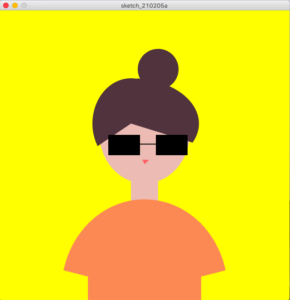Motivation

Me wearing sunglasses in the Abu Dhabi’s sun (thus yellow background!)

Process

The main purpose of the assignment is to get myself accustomed to the use of some functions in drawing shapes and lines. I found myself struggling with the arc function when drawing the bang and the shoulders.

The hair still does not look symmetrical but I am happy with it. I intended to draw untied hair at first as that’s how I usually look like, by putting 2 straight lines starting from my head to my shoulders. However, I could not find the shape I was satisfied with to fill in the hair color as well. I was wondering if we could fill in color within an area restricted by boundaries (not necessarily shapes). Eventually, I finished with a hair bun by drawing a circle which turns out quite suitable with the overall hot season and my initial motivation.

Regarding the shoulders, I attempted to use 2 separate arc functions at first to make them look smoother than a rectangle but the symmetry didn’t work well. I decided to go with one single arc instead and it worked!

```void setup() {
size (640, 640);
};

void draw() {
background (255,255,0);

int x = width/2;
int y = height/2;

//face
noStroke();
fill(236,188,180);
ellipse(x,y-50,200,225);

//torso
rect(x-30,y+57,60,43);
fill(252,137,83);
rect(x-125,y+150,250,300);

//hair
fill(80,51,60);
circle(x+30,y-190,90);

//sunglasses
fill(0);
rect(x-80,y-45,70,45);
rect(x+25,y-45,70,45);
stroke(0);
strokeWeight(2);
line(x-80,y-25,x+25,y-25);

//mouth
noStroke();
fill(252,98,104);
triangle(x-5,y+10,x,y+20,x+10,y+10);
}

```